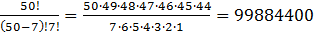### Sample Problem

In the LuckyLulu Lottery, a player chooses seven different numbers from 1 to 50. If these seven numbers match the seven numbers drawn (in any order) by the LuckyLulu lottery commission, the player wins. What is the probability of the player winning if she buys one ticket?

#### Solution

To find the number of elements in the sample space, use the formula for the number of combinations of 50 elements taken seven at a time.

n(S)=50C7=So, if the player buys only one ticket, her probability of winning is